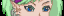-=+=- -=+=- -=+=- -=+=- -=+=- -=+=- -=+=- -=+=- -=+=- -=+=- -=+=- -=+=- -=+=- -=+=- -=+=- -=+=- -=+=- -=+=- -=+=- -=+=- -=+=- -=+=- -=+=- -=+=- -=+=- -=+=- -=+=- -=+=- -=+=- -=+=- (c) WidthPadding Industries 1987 0|330|0 -=+=- -=+=- -=+=- -=+=- -=+=- -=+=- -=+=- -=+=- -=+=- -=+=- -=+=- -=+=- -=+=- -=+=- -=+=- -=+=- -=+=- -=+=- -=+=- -=+=- -=+=- -=+=- -=+=- -=+=- -=+=- -=+=- -=+=- -=+=- -=+=- -=+=- Socoder -> Off Topic -> RIP : Steve's Uncle Posted : Monday, 09 December 2019, 09:03 steve_ancellJust found out that my uncle died this morning, fuck shit bollocks! Posted : Monday, 09 December 2019, 09:03 JayenkaiAwwcommiserationsPosted : Monday, 09 December 2019, 09:16 PakzMy condoleances! My uncle has alzheimer. We might loose him in a couple of years also.Posted : Monday, 09 December 2019, 09:27 JayenkaiYeah. I meant that word. I always (ALWAYS) get that wrong.. Was he much older? -=-=- ''Load, Next List!''Posted : Monday, 09 December 2019, 11:02 steve_ancellHis name is Jim my, he my dad's brother. He was 80 years old but had all of his marbles. Someone found him in a corridor at the sheltered flats he lives at, I'm shocked, my cousin is in a right mess too. Posted : Monday, 09 December 2019, 11:05 JayenkaiAww, fuck.Posted : Monday, 09 December 2019, 15:07 rockfordMy condolences to you and the rest of your family.R.I.P. Posted : Tuesday, 10 December 2019, 13:03 TrickyMy condolences...Posted : Tuesday, 10 December 2019, 14:10 spinalI think all our thoughts are with you Steve.Posted : Tuesday, 10 December 2019, 17:29 steve_ancellThanks guys.